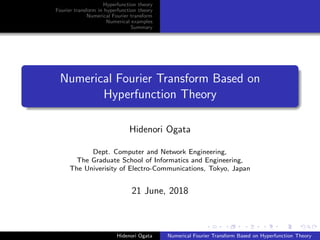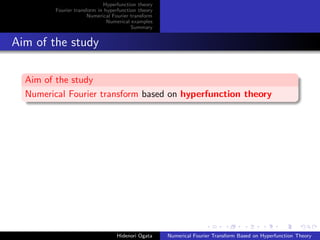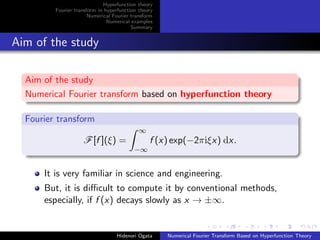Successfully reported this slideshow.

# Numerical Fourier transform based on hyperfunction theory×

# Numerical Fourier transform based on hyperfunction theory

This is the PC slide of a contributed talk in the conference "ECMI2018 (The 20th European Conference on Mathematics for Industry)", 18-20 June 2018, Budapest, Hungary. In this talk, we propose a numerical method of Fourier transforms based on hyperfunction theory.

This is the PC slide of a contributed talk in the conference "ECMI2018 (The 20th European Conference on Mathematics for Industry)", 18-20 June 2018, Budapest, Hungary. In this talk, we propose a numerical method of Fourier transforms based on hyperfunction theory.

### Numerical Fourier transform based on hyperfunction theory

1. 1. Hyperfunction theory Fourier transform in hyperfunction theory Numerical Fourier transform Numerical examples Summary Numerical Fourier Transform Based on Hyperfunction Theory Hidenori Ogata Dept. Computer and Network Engineering, The Graduate School of Informatics and Engineering, The Univerisity of Electro-Communications, Tokyo, Japan 21 June, 2018 Hidenori Ogata Numerical Fourier Transform Based on Hyperfunction Theory
2. 2. Hyperfunction theory Fourier transform in hyperfunction theory Numerical Fourier transform Numerical examples Summary Aim of the study Aim of the study Numerical Fourier transform based on hyperfunction theory Hidenori Ogata Numerical Fourier Transform Based on Hyperfunction Theory
3. 3. Hyperfunction theory Fourier transform in hyperfunction theory Numerical Fourier transform Numerical examples Summary Aim of the study Aim of the study Numerical Fourier transform based on hyperfunction theory Fourier transform F[f ](ξ) = ∞ −∞ f (x) exp(−2πiξx) dx. It is very familiar in science and engineering. But, it is diﬃcult to compute it by conventional methods, especially, if f (x) decays slowly as x → ±∞. Hidenori Ogata Numerical Fourier Transform Based on Hyperfunction Theory
4. 4. Hyperfunction theory Fourier transform in hyperfunction theory Numerical Fourier transform Numerical examples Summary Fourier transforms are diﬃcult to compute! Numerical integration by the DE rule. (1) ∞ 0 dx 1 + x2 , (2) ∞ 0 cos x 1 + x2 dx. -16 -14 -12 -10 -8 -6 -4 -2 0 0 10 20 30 40 50 60 70 log10(error) N (1) (2) vertical axis: log10(error) horizontal axis: number of sampling points N Hidenori Ogata Numerical Fourier Transform Based on Hyperfunction Theory
5. 5. Hyperfunction theory Fourier transform in hyperfunction theory Numerical Fourier transform Numerical examples Summary Aim of the study Aim of the study Numerical Fourier transform based on hyperfunction theory Fourier transform F[f ](ξ) = ∞ −∞ f (x) exp(−2πiξx) dx. It is very familiar in science and engineering. But, it is diﬃcult to compute it by conventional methods, especially, if f (x) decays slowly as x → ±∞. It is easy to compute it using hyperfunction theory. Hidenori Ogata Numerical Fourier Transform Based on Hyperfunction Theory
6. 6. Hyperfunction theory Fourier transform in hyperfunction theory Numerical Fourier transform Numerical examples Summary Contents Contents 1 Basis of hyperfunction theory 2 Fourier transform in hyperfunction theory 3 Numerical Fourier transform 4 Numerical examples 5 Summary Hidenori Ogata Numerical Fourier Transform Based on Hyperfunction Theory
7. 7. Hyperfunction theory Fourier transform in hyperfunction theory Numerical Fourier transform Numerical examples Summary 1. Hyperfunction theory M. Sato, 1958 a theory of generalized functions based on complex function theory Hyperfunction the diﬀerence between the boundary values of an analytic function F(z) f (x) = [F(z)] ≡ F(x + i0) − F(x − i0). F(z) : deﬁning function of the hyperfunction f (x) Hidenori Ogata Numerical Fourier Transform Based on Hyperfunction Theory
8. 8. Hyperfunction theory Fourier transform in hyperfunction theory Numerical Fourier transform Numerical examples Summary 1. Hyperfunction: f (x) = F(x + i0) − F(x − i0) Examples δ(x) = − 1 2πi 1 x + i0 − 1 x − i0 = lim ǫ↓0 1 π ǫ x2 + ǫ2 , χ(−1,1)(x) = 1 x ∈ (−1, 1) 0 x ∈ [−1, 1] = − 1 2πi log (x + i0) + 1 (x + i0) − 1 − log (x − i0) + 1 (x − i0) − 1 . log z: the principal value, i.e., log x ∈ R for x > 0. -1 -0.5 0 0.5 1Re z -1 -0.5 0 0.5 1 Im z -6 -4 -2 0 2 4 6 Re F(z) -2 -1.5 -1 -0.5 0 0.5 1 1.5 2Re z -2-1.5-1-0.500.511.52 Im z -0.6 -0.4 -0.2 0 0.2 0.4 0.6 Re F(z) deﬁning function F(z) of δ(x) deﬁning function F(z) of χ(−1,1)(x) Hidenori Ogata Numerical Fourier Transform Based on Hyperfunction Theory
9. 9. Hyperfunction theory Fourier transform in hyperfunction theory Numerical Fourier transform Numerical examples Summary 2. Fourier transform in hyperfunction theory How to treat Fourier transforms in hyperfunction theory. F[f ](ξ) = ∞ −∞ f (x)e−2πiξx dx. Hidenori Ogata Numerical Fourier Transform Based on Hyperfunction Theory
10. 10. Hyperfunction theory Fourier transform in hyperfunction theory Numerical Fourier transform Numerical examples Summary 2. Fourier transform in hyperfunction theory How to treat Fourier transforms in hyperfunction theory. F[f ](ξ) = 0 −∞ f (x)e−2πi(ξ+i0)x dx + +∞ 0 f (x)e−2πi(ξ−i0)x dx . We can deﬁne F[f ](ξ) thanks to e−2πǫ|x| even if the integral is not convergent in the conventional sense. Hidenori Ogata Numerical Fourier Transform Based on Hyperfunction Theory
11. 11. Hyperfunction theory Fourier transform in hyperfunction theory Numerical Fourier transform Numerical examples Summary 2. Fourier transform in hyperfunction theory How to treat Fourier transforms in hyperfunction theory. F[f ](ξ) = 0 −∞ f (x)e−2πi(ξ+i0)x dx + +∞ 0 f (x)e−2πi(ξ−i0)x dx . We can deﬁne F[f ](ξ) thanks to e−2πǫ|x| even if the integral is not convergent in the conventional sense. (Example) F(ξ) = 0 −∞ e−2πi(ξ+i0)x dx + ∞ 0 e−2πi(ξ−i0)x dx = − 1 2πi 1 ξ + i0 − 1 ξ − i0 = δ(ξ). Hidenori Ogata Numerical Fourier Transform Based on Hyperfunction Theory
12. 12. Hyperfunction theory Fourier transform in hyperfunction theory Numerical Fourier transform Numerical examples Summary 2. Fourier transform in hyperfunction theory How to treat Fourier transforms in hyperfunction theory. F[f ](ξ) = 0 −∞ f (x)e−2πi(ξ+i0)x dx + +∞ 0 f (x)e−2πi(ξ−i0)x dx . We can deﬁne F[f ](ξ) thanks to e−2πǫ|x| even if the integral is not convergent in the conventional sense. Fourier transform F[f ](ξ) Hyperfunction with the deﬁning functions F±(ζ) F[f ](ξ) = F+(ξ + i0) − F−(ξ − i0), F+(ζ) = 0 −∞ f (x)e−2πiζx dx ( Im ζ > 0 ), F−(ζ) = − ∞ 0 f (x)e−2πiζx dx ( Im ζ < 0 ). Hidenori Ogata Numerical Fourier Transform Based on Hyperfunction Theory
13. 13. Hyperfunction theory Fourier transform in hyperfunction theory Numerical Fourier transform Numerical examples Summary 3. Numerical Fourier transform: strategy We use the deﬁnition of Fourier transforms in hyperfunction theory for numerical Fourier transforms. F[f ](ξ) = ∞ −∞ f (x)e−2πiξx dx = F+(ξ + i0) − F−(ξ − i0), where F+(ζ) = 0 −∞ f (x)e−2πiζx dx ( Im ζ > 0 ), F−(ζ) = − ∞ 0 f (x)e−2πiζx dx ( Im ζ < 0 ). We can compute F±(ζ) in C because the integrands include the exponentially decaying factor exp(−2π| Im ζ||x|). Hidenori Ogata Numerical Fourier Transform Based on Hyperfunction Theory
14. 14. Hyperfunction theory Fourier transform in hyperfunction theory Numerical Fourier transform Numerical examples Summary 3. Numerical Fourier transform: strategy We use the deﬁnition of Fourier transforms in hyperfunction theory for numerical Fourier transforms. F[f ](ξ) = ∞ −∞ f (x)e−2πiξx dx = F+(ξ + i0) − F−(ξ − i0), where F+(ζ) = 0 −∞ f (x)e−2πiζx dx ( Im ζ > 0 ), F−(ζ) = − ∞ 0 f (x)e−2πiζx dx ( Im ζ < 0 ). Strategy 1 Get F±(ζ) in { ζ ∈ C | ± Im ζ > 0 }. 2 Get F±(ξ ± i0) on R by analytic continuation. Hidenori Ogata Numerical Fourier Transform Based on Hyperfunction Theory
15. 15. Hyperfunction theory Fourier transform in hyperfunction theory Numerical Fourier transform Numerical examples Summary 3. Numerical Fourier transform: strategy 1/2 1. Get F±(ζ) in { ζ ∈ C | ± Im ζ > 0 }. F+(ζ) = 0 −∞ f (x)e−2πiζx dx ( Im ζ > 0 ), F−(ζ) = − ∞ 0 f (x)e−2πiζx dx ( Im ζ < 0 ). Hidenori Ogata Numerical Fourier Transform Based on Hyperfunction Theory
16. 16. Hyperfunction theory Fourier transform in hyperfunction theory Numerical Fourier transform Numerical examples Summary 3. Numerical Fourier transform: strategy 1/2 1. Get F±(ζ) in { ζ ∈ C | ± Im ζ > 0 }. F±(ζ) = ± ∞ 0 f (∓x)e±2πiζx dx ( ± Im ζ > 0 ). Hidenori Ogata Numerical Fourier Transform Based on Hyperfunction Theory
17. 17. Hyperfunction theory Fourier transform in hyperfunction theory Numerical Fourier transform Numerical examples Summary 3. Numerical Fourier transform: strategy 1/2 1. Get F±(ζ) in { ζ ∈ C | ± Im ζ > 0 }. F±(ζ) = ± ∞ 0 f (∓x)e±2πiζx dx ( ± Im ζ > 0 ). We get F±(ζ) in Taylor series. F±(ζ) = ∞ n=0 c (±) n (ζ − ζ (±) 0 )n ( ± Im ζ (±) 0 > 0 ), c (±) n = 1 n! F (n) ± (ζ (±) 0 ) = ± 1 n! ∞ 0 (±2πix)n f (∓x)e±2πiζ (±) 0 x exponential decay dx. Hidenori Ogata Numerical Fourier Transform Based on Hyperfunction Theory
18. 18. Hyperfunction theory Fourier transform in hyperfunction theory Numerical Fourier transform Numerical examples Summary 3. Numerical Fourier transform: strategy 2/2 2. Get F±(ξ ± i0) on R by analytic continuation. Taylor series → continued fraction F±(ζ) = ∞ n=0 cn(ζ − ζ (±) 0 )n = a (±) 0 1 + a (±) 1 (ζ − ζ (±) 0 ) 1 + a (±) 2 (ζ − ζ (±) 0 ) 1 + ... R R ζ (±) 0 convergence region c (±) n → a (±) n by quotient diﬀerence (QD) algorithm The QD algorithm is unstable. → multiple precision arithmetic Hidenori Ogata Numerical Fourier Transform Based on Hyperfunction Theory
19. 19. Hyperfunction theory Fourier transform in hyperfunction theory Numerical Fourier transform Numerical examples Summary 3. Numerical Fourier transform: strategy 2/2 2. Get F±(ξ ± i0) on R by analytic continuation. Taylor series → continued fraction F±(ζ) = ∞ n=0 cn(ζ − ζ (±) 0 )n = a (±) 0 1 + a (±) 1 (ζ − ζ (±) 0 ) 1 + a (±) 2 (ζ − ζ (±) 0 ) 1 + ... R R ζ (±) 0 convergence region F[f ](ξ) = F+(ξ + i0) − F−(ξ − i0). Hidenori Ogata Numerical Fourier Transform Based on Hyperfunction Theory
20. 20. Hyperfunction theory Fourier transform in hyperfunction theory Numerical Fourier transform Numerical examples Summary 4. Numerical examples (1) F[tanh(πx)](ξ) = −i cosech(πξ), (2) F[(1 + x2 )−ν−1/2 ] = 2πν+1/2 Γ(ν + 1/2) |ξ|ν Kν(2π|ξ|) ( ν = 1.5 ), (3) F[log |x|](ξ) = −γδ(ξ) − 1 2|ξ| . The center of the Taylor series of F±(ζ): ζ (±) 0 = ±i. C++ programs, multiple precision arithmetic (100 decimal digits). exﬂib: multiple precision arithmetic library. Hidenori Ogata Numerical Fourier Transform Based on Hyperfunction Theory
21. 21. Hyperfunction theory Fourier transform in hyperfunction theory Numerical Fourier transform Numerical examples Summary 4. Numerical examples: errors of our method -60 -50 -40 -30 -20 -10 0 -4 -2 0 2 4 log10(error) xi (1) (2) (3) vertical axis: log10(error) horizontal axis: ξ (1) F[tanh(πx)](ξ), (2) F[(1+x2 )−ν−1/2 ](ξ), (3) F[log |x|]. The number of sampling points for numerical integration (the DE rule) (1) 1330 (2) 1416 (3) 1430. Hidenori Ogata Numerical Fourier Transform Based on Hyperfunction Theory
22. 22. Hyperfunction theory Fourier transform in hyperfunction theory Numerical Fourier transform Numerical examples Summary 4. Numerical examples: an interesting fact We do not need to compute oscillatory integrals for Fourier transforms. Hidenori Ogata Numerical Fourier Transform Based on Hyperfunction Theory
23. 23. Hyperfunction theory Fourier transform in hyperfunction theory Numerical Fourier transform Numerical examples Summary 4. Numerical examples: an interesting fact We do not need to compute oscillatory integrals for Fourier transforms. The deﬁning function ( F[f ](ξ) = F+(ξ + i0) − F−(ξ − i0) ) F±(ζ) = ± ∞ 0 f (∓x)e±2πiζx dx = ∞ n=0 c (±) n (ζ ∓ i)n ( ± Im ζ > 0 ), c (±) n = ± 1 n! ∞ 0 (±2πix)n f (∓x) exp(−2πx)dx. including no oscillatory function. Hidenori Ogata Numerical Fourier Transform Based on Hyperfunction Theory
24. 24. Hyperfunction theory Fourier transform in hyperfunction theory Numerical Fourier transform Numerical examples Summary 4. Numerical examples: comparisons Comparison with the previous methods 1 DE rule & Richardson extrapolation M. Sugihara, J. Comp. Appl. Math., 17 (1987) 47–68. F[f ](ξ) = lim n→∞ ∞ −∞ f (x) exp(−2πiξx)exp(−2−n x2 )dx. 2 DE-type rule for oscillatory integrals T. Ooura & M. Mori, J. Comp. Appl. Math., 38 (1991) 353–360. Hidenori Ogata Numerical Fourier Transform Based on Hyperfunction Theory
25. 25. Hyperfunction theory Fourier transform in hyperfunction theory Numerical Fourier transform Numerical examples Summary 4. Numerical examples: comparisons F[tanh(πx)](ξ) = −i cosech(πξ), ξ = 1. multiple precision arithmetic (100 decimal digits, exﬂib) the center of the Taylor series ζ (±) 0 = 1 ± i. Hidenori Ogata Numerical Fourier Transform Based on Hyperfunction Theory
26. 26. Hyperfunction theory Fourier transform in hyperfunction theory Numerical Fourier transform Numerical examples Summary 4. Numerical examples: comparisons F[tanh(πx)](ξ) = −i cosech(πξ), ξ = 1. multiple precision arithmetic (100 decimal digits, exﬂib) the center of the Taylor series ζ (±) 0 = 1 ± i. number of sampling points for integration error our method 2610 2.8 × 10−28 DE & Richardson 10060 9.1 × 10−20 DE for oscillatory integrals 948 5.0 × 10−25 Hidenori Ogata Numerical Fourier Transform Based on Hyperfunction Theory
27. 27. Hyperfunction theory Fourier transform in hyperfunction theory Numerical Fourier transform Numerical examples Summary 4. Numerical examples: comparisons number of sampling points for integration error our method 2610 2.8 × 10−28 DE & Richardson 10060 9.1 × 10−20 DE for oscillatory integrals 948 5.0 × 10−25 Hidenori Ogata Numerical Fourier Transform Based on Hyperfunction Theory
28. 28. Hyperfunction theory Fourier transform in hyperfunction theory Numerical Fourier transform Numerical examples Summary 4. Numerical examples: comparisons number of sampling points for integration error our method 2610 2.8 × 10−28 DE & Richardson 10060 9.1 × 10−20 DE for oscillatory integrals 948 5.0 × 10−25 Our method > DE & Richardson Our method < DE for oscillatory integrals Our method computes F[f ](ξ) as a function, i.e., once we obtain the coeﬃcients a (±) n of the continued fraction, we can compute F[f ](ξ)’s for many ξ’s. The conventional methods compute F[f ](ξ) as an integrals. Hidenori Ogata Numerical Fourier Transform Based on Hyperfunction Theory
29. 29. Hyperfunction theory Fourier transform in hyperfunction theory Numerical Fourier transform Numerical examples Summary Summary 1 Numerical Fourier transform based on hyperfunction theory 2 Fourier transform as a hyperfunction F[f ](ξ) = 0 −∞ f (x)e−2πi(ξ+i0)x dx + ∞ 0 f (x)e−2πi(ξ−i0)x dx. deﬁning functions F±(ζ) Get F±(ζ) in { ζ ∈ C | ± Im ζ > 0 }. Get F±(ξ ± i0) on R by analytic continuation (by the continued fraction). 3 Numerical examples shows the eﬀectiveness of our method. Problems for future studies 1 Theoretical error estimate 2 Analytic continuation by continued fractions Hidenori Ogata Numerical Fourier Transform Based on Hyperfunction Theory
30. 30. Hyperfunction theory Fourier transform in hyperfunction theory Numerical Fourier transform Numerical examples Summary Thank you very much! Hidenori Ogata Numerical Fourier Transform Based on Hyperfunction Theory